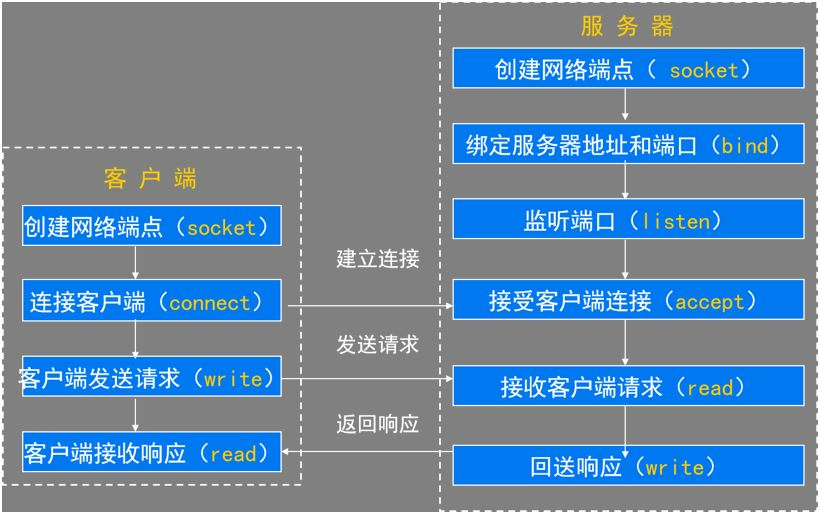# python实现socket通信

(在苹果系统下，如果文章中的图片不能正常显示，请升级Safari浏览器到最新版本，或者使用Chrome、Firefox浏览器打开。)

python可以用来实现两个主机进程之间的TCP通信，就是通过使用socket套接字来实现的，我们可以基于此，来实现一些应用层协议，以达到不同主机之间的进程通信的目的。这一点，除了普通的网络应用程序（如聊天工具和游戏等）之外，在分布式系统中，也有着很大的用途，比如机器学习需要在多台计算机中进行并行计算的时候。TCP服务器/客户端模型

1 循环服务器

2 并发服务器

```import multiprocessing
import socket

class ClientProcess(multiprocessing.Process):
def __init__(self,conn,addr):
multiprocessing.Process.__init__(self)
self.conn = conn
self.addr = addr

def run(self):
self.conn.sendall(bytes("你好，我是AI柠檬~",encoding="utf-8"))
ret_timeout = str(self.conn.recv(1024),encoding="utf-8")
self.conn.settimeout(int(ret_timeout))

while(True):
try:
ret = str(self.conn.recv(1024),encoding="utf-8")
self.conn.sendall(bytes("我收到了你的信息："+ret,encoding="utf-8"))
if(ret==":q"):
self.conn.close()
break
except Exception as ex:
self.conn.close()
break

if __name__ == '__main__':
s = socket.socket()
inp_addr = input("Please input this server's ip address:\n>>>")
inp_port = input("Please input this server's port:\n>>>")
s.bind((inp_addr,int(inp_port)))
s.listen()
print("服务器已开启")
users = []
while(True):
conn,addr=s.accept()

p = ClientProcess(conn,addr)
p.start()
users.append(p)
print("有一个新用户连接，进程号pid:", p.pid)
num = 0

tmp_users=[]
for i in range(len(users)):

if(users[i].is_alive()==True):
tmp_users.append(users[i])

users = tmp_users
print("当前用户数：", len(users))
s.close()
```

```import socket

obj = socket.socket()

inp_addr = input("Please input server's ip address:\n>>>")
inp_port = input("Please input server's port:\n>>>")
obj.connect((inp_addr,int(inp_port)))

inp_timeout = input("Please input timeout(s):\n>>>")
obj.sendall(bytes(inp_timeout,encoding="utf-8"))
obj.settimeout(int(inp_timeout))

msg = str(obj.recv(1024),encoding="utf-8")
print(msg)

while True:
inp = input("Please(:q\退出):\n>>>")
if(inp!=""):
obj.sendall(bytes(inp,encoding="utf-8"))
if(inp == ":q"):
obj.close()
break
try:
ret = str(obj.recv(1024),encoding="utf-8")
print(ret)
except socket.timeout:
print("[Error]Network Connection Time Out!")
break
except:
print("[Error]Unknown Network Connection Error!")
break

```

 版权声明本博客的文章除特别说明外均为原创，本人版权所有。欢迎转载，转载请注明作者及来源链接，谢谢。本文地址: https://blog.ailemon.me/2018/01/21/python-implement-about-socket-connection/ All articles are under Attribution-NonCommercial-ShareAlike 4.0